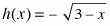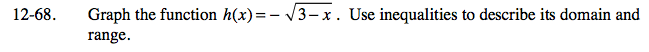### Home > CA > Chapter Ch12 > Lesson 12.4.1 > Problem12-68

12-68.

Graph the function. Use inequalities to describe its domain and range. Homework Help ✎Make a table and plot points to help you graph the equation.

What are the possible x-values, or the domain of the function?

What are the possible y-values, or the range of the function?